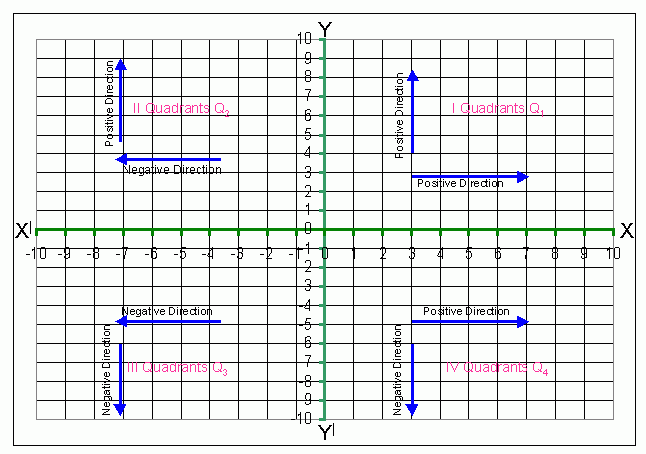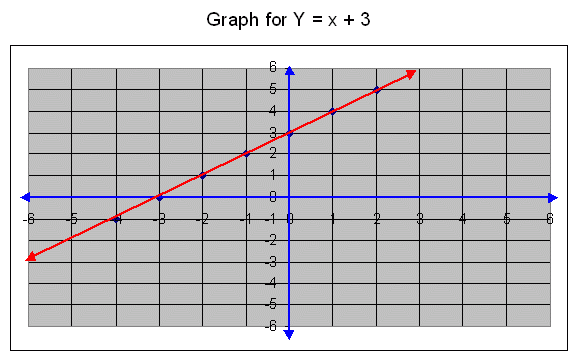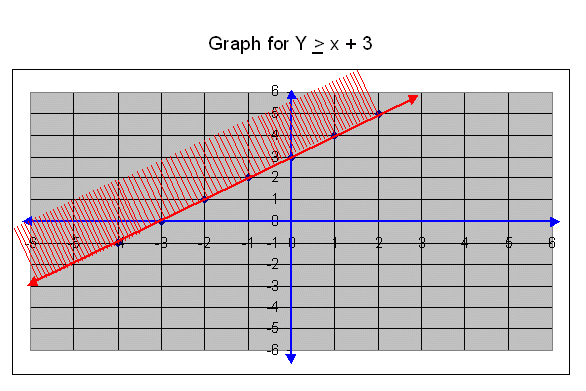Email us to get an instant 20% discount on highly effective K-12 Math & English kwizNET Programs!

#### Online Quiz (WorksheetABCD)

Questions Per Quiz = 2 4 6 8 10

### High School Mathematics - 29.1 General Concepts - Coordinate Geometry or Cartesian C or Analytical Geometry

In a coordinate plane the horizontal line is called the x-axis and the vertical line is called the y-axis. The point of intersection of these axes is called the origin.In the above diagram, X and Y axes divided the plane into four regions and these regions are called Quadrants and they are numbered Q1, Q2, Q3 and Q4, in the anti clock wise direction the first quadrant is denoted as Q1, the second quadrant is denoted as Q2, the third quadrant as Q3 and the fourth quadrant as Q4.

The right hand side towards east form the Y-axis is considered as positive direction. The left hand side towards west from the Y-axis is considered as negative directions. The upward direction towards north from X-axis is positive direction and the downward side towards the south is negative direction.

In the first quadrant Q1 the coordinates x and y are (+,+). That is a positive real number and a positive real number.
In the second quadrant Q2 the coordinates x and y are (-,+). That is a negative real number and a positive real number.
In the third quadrant Q3 the coordinates x and y are (-,-). That is a negative real number and a negative real number.
In the first quadrant Q4 the coordinates x and y are (+,-). That is a positive real number and a negative real number.

#### Plotting of Points on a Graph PaperIn the above graph the point P is at the distance of 3 units from Y-axis and 4 units from X-axis. The ordered pair corresponding to the point P is (3,4).
The ordered pair (3,4), which denote the point P. In this 3 is called as the first coordinate and 4 is called second coordinate. The first coordinate 3 is known as x-coordinate and second coordinate 4 is known as y-coordinate.
The point Q is at a distance of 5 units from Y-axis and it is at a distance of 3 units from X-axis the ordered pair corresponding to the point Q is (5,3).

If we observe the points A (2,3) and B is (3,2) in the above graph, the ordered pairs of A and B denote two different points. Hence (2,3) is not equal to (3,2).

Plotting of negative coordinates:
Plot S(2,-3), we start from the origin proceed 2 units towards right hand side of the origin, and from their we start 3 units downwards that is negative direction to reach the point S.
In the same way we have plotted the points T(-2,-4) and U(-5,4).

#### Graph For Equation

Plot the following points on a graph sheet
(-4,0),(-3,0),(-2,0),(-1,0),(0,0),(1,0),(2,0),(3,0),(4,0).Observing the above graph:

1. All points lie on X-axis.
2. The common property of all these points is its y-coordinate is zero.
3. The straight line joining all these points may be denoted as y=0.

The y-coordinate on X-axis is zero. X-axis may be denoted by the straight line y=o.

Plot the following points on a graph sheet.
(0,-1),(0,-2),(0,-3,),(0,-4),(0,0),(0,1),(0,2),(0,3),(0,4)Observing the above graph:

1. All these points lie on Y-axis.
2. The common property of all these points is x = o.
3. The x-coordinate of any point on y-axis is zero.
4. We can denote the y-axis as x=0.

The x-coordinate on Y-axis is zero. Y-axis may be denoted by the straight line x=o.

#### Graph For Inequations

Draw a graph for y ł x + 3.

First we draw a straight line for y = x + 3.

The ordered pairs for the graph y = x+3.
x-4-3-2-1012
y = x + 3-1012345
Ordered pairs(-4,-1)(-3,0)(-2,1)(-1,2)(0,3)(1,4)(2,5)To find out the region for y ł x + 3, first we find out that the origin lies in the region represented by the graph or not.
We substitute the coordinates of the origin (0,0) in the given inequation,
y ł x + 3, we get,
0 ł 0 + 3
0 ł 3, this is wrong.
The origin does not lie in the half plane represented the graph.
Graph for y ł x + 3, isThe boundary line y = x + 3 lies in the region represented by above graph. The boundary line is drawn as a dark line. The region indicated by the graph is shaded.

Its solution set is {(x,y)/(x,y) Î R and y ł x + 3}